## Deconstruct Multichannel Signals for Frame-Based Processing

In frame-based processing, blocks and System objects process data in frames. Each frame of data contains samples of consecutive times stacked together. Each channel is represented by a column of the input signal. For example, from a frame-based processing perspective, this 3-by-2 matrix has two channels, each containing three samples.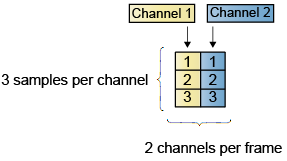When you configure a block to perform frame-based processing, the block interprets an M-by-1 vector as a single-channel signal containing M samples per frame. Similarly, the block interprets an M-by-N matrix as a multichannel signal with N independent channels and M samples per channel. For example, in frame-based processing, blocks interpret this sequence of 3-by-2 matrices as a two-channel signal with a frame size of 3.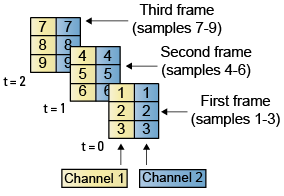### Split Multichannel Signals into Individual Signals

Multichannel signals, represented by matrices in the Simulink® environment, are frequently used in signal processing models for efficiency and compactness. Though most of the signal processing blocks can process multichannel signals, you may need to access just one channel or a particular range of samples in a multichannel signal. You can access individual channels of the multichannel signal by using the blocks in the Indexing library. This library includes the Selector, Submatrix, Variable Selector, Multiport Selector, and Submatrix blocks. It is also possible to use the Permute Matrix block, in the Matrix operations library, to reorder the channels of a frame-based signal.

You can use the Multiport Selector block in the Indexing library to extract the individual channels of a multichannel signal. These signals form single-channel signals that have the same frame rate and frame size of the multichannel signal.

The figure below is a graphical representation of this process.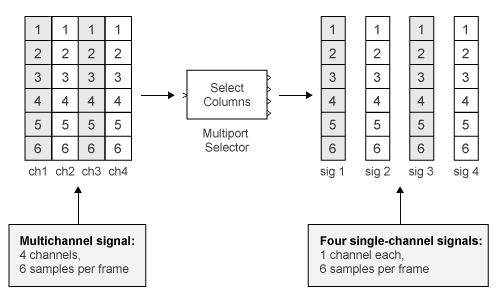In this example, you use the Multiport Selector block to extract a single-channel signal and a two channel signal from a multichannel signal. Each channel contains four samples.

Open the `ex_splitmltichfbsigsind` model.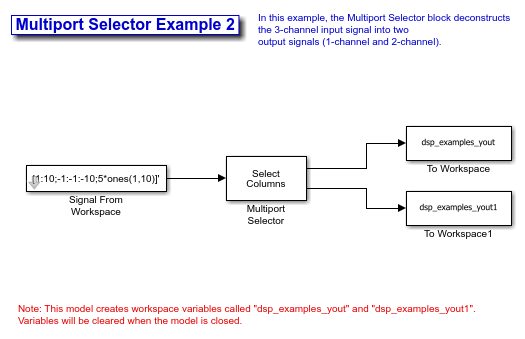Double-click the Signal From Workspace block, and set the block parameters as follows:

• Signal = [1:10;-1:-1:-10;5*ones(1,10)]'

• Samples per frame = 4

Based on these parameters, the Signal From Workspace block outputs a three-channel signal with a frame size of four. Save these parameters and close the dialog box by clicking OK.

Double-click the Multiport Selector block. Set the block parameters as follows, and then click OK:

• Select = `Columns`

• Indices to output = {[1 3],2}

Based on these parameters, the Multiport Selector block outputs the first and third columns at the first output port and the second column at the second output port of the block. Setting the Select parameter to `Columns` ensures that the block preserves the frame rate and frame size of the input.

Run the model. The figure below is a graphical representation of how the Multiport Selector block splits one frame of the three-channel signal into a single-channel signal and a two-channel signal.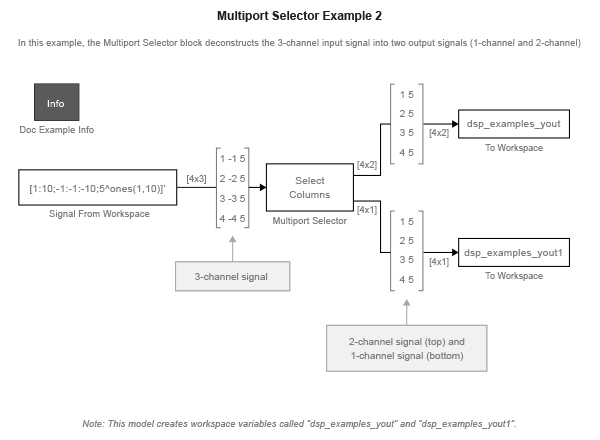The Multiport Selector block outputs a two-channel signal, comprised of the first and third column of the input signal, at the first port. It outputs a single-channel comprised of the second column of the input signal, at the second port.

You have now successfully created a single-channel signal and a two-channel signal from a multichannel signal using the Multiport Selector block.

### Reorder Channels in Multichannel Signals

Multichannel signals, represented by matrices in Simulink are frequently used in signal processing models for efficiency and compactness. Though most of the signal processing blocks can process multichannel signals, you may need to access just one channel or a particular range of samples in a multichannel signal. You can access individual channels of the multichannel signal by using the blocks in the Indexing library. This library includes the Selector, Submatrix, Variable Selector, Multiport Selector, and Submatrix blocks. It is also possible to use the Permute Matrix block, in the Matrix operations library, to reorder the channels of a multichannel signal.

Some DSP System Toolbox™ blocks have the ability to process the interaction of channels. Typically, DSP System Toolbox blocks compare channel one of signal A to channel one of signal B. However, you might want to correlate channel one of signal A with channel three of signal B. In this case, in order to compare the correct signals, you need to use the Permute Matrix (Simulink) block to rearrange the channels of your signals. This example explains how to accomplish this task.

Open the `ex_reordermltichfbsigs` model.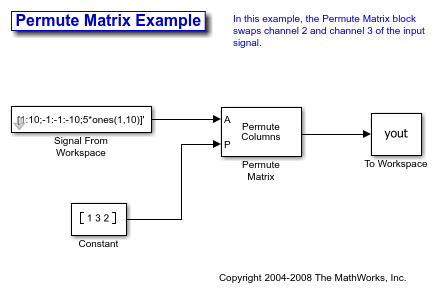Double-click the Signal From Workspace block, and set the block parameters as follows:

• Signal = [1:10;-1:-1:-10;5*ones(1,10)]'

• Sample time = 1

• Samples per frame = 4

Based on these parameters, the Signal From Workspace block outputs a three-channel signal with a sample period of 1 second and a frame size of 4. The frame period of this block is 4 seconds.

Save these parameters and close the dialog box by clicking OK.

Double-click the Constant block. Set the block parameters as follows, and then click OK:

• Constant value = [1 3 2] Clear the Interpret vector parameters as 1-D check box

• Sample time = 4

The discrete-time vector output by the Constant block tells the Permute Matrix block to swap the second and third columns of the input signal. Note that the frame period of the Constant block must match the frame period of the Signal From Workspace block.

Double-click the Permute Matrix block. Set the block parameters as follows, and then click OK:

• Permute = `Columns`

• Index mode = `One-based`

Based on these parameters, the Permute Matrix block rearranges the columns of the input signal, and the index of the first column is now one.

Run the model. The figure below is a graphical representation of what happens to the first input frame during simulation.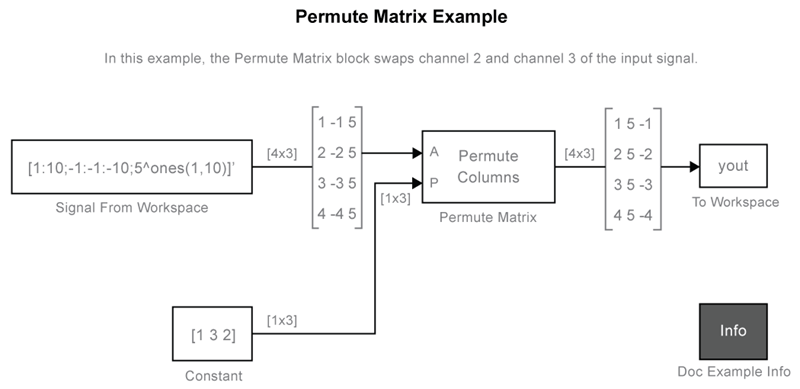The second and third channel of the input signal are swapped.

At the MATLAB command line, type `yout`.

```yout = 1 5 -1 2 5 -2 3 5 -3 4 5 -4 5 5 -5 6 5 -6 7 5 -7 8 5 -8 9 5 -9 10 5 -10 0 0 0 0 0 0 ```

You can now verify that the second and third columns of the input signal are rearranged.

You have now successfully reordered the channels of a signal using the Permute Matrix block.